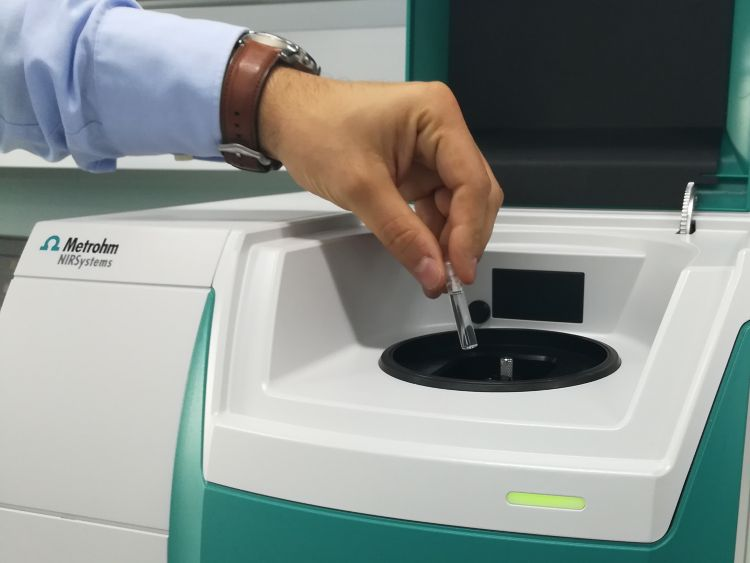You have been redirected to your local version of the requested page

Determination of the acid concentration in mixed acid solutions is a critical quality control step for successful etching processes. While primary analytical methods such as thermometric titration are well known, difficulties arise when mixtures of three or more acids need to be analyzed or if the time to result is a critical aspect. This application note discusses an alternative near-infrared (NIR) spectroscopy method that can reliably determine all parameters within a minute.Figure 1. DS2500 Liquid Analyzer and a sample filled in a disposable vial.

Mixed acid solutions based on four different acids (H3PO4, H2SO4, HNO3, and HF) were measured in transmission mode with a DS2500 Liquid Analyzer over the full wavelength range (400–2500 nm). Disposable vials with a pathlength of 2 mm were used for convenient and fast measurement. The Metrohm software package Vision Air Complete was used for all data acquisition and prediction model development.

Table 1. Hardware and software equipment overview
Equipment Metrohm number
DS2500 Liquid Analyzer 2.929.0010
DS2500 Holder 2 mm vials 6.7492.000
Disposable vials, 2 mm diameter, transmission 6.7402.070
Vision Air 2.0 Complete 6.6072.208

27 measured Vis-NIR spectra (Figure 2) were used to create a prediction model for quantification of the different acid concentrations (H3PO4, H2SO4, HNO3, and HF). The quality of the prediction models was evaluated using correlation diagrams, which show a very high correlation between Vis-NIR prediction and primary method values. The respective figures of merit (FOM) display the expected precision of a prediction during routine analysis.

Figure 2. Vis-NIR spectra of mixed acids solutions with varying acid content measured on a DS2500 Liquid Analyzer. For display reasons a spectra offset was applied.

### Result H3PO4

Figure 3. Correlation diagram for the prediction of H3PO4 content in a mixed acid solution using a DS2500 Liquid Analyzer.
Table 2. Figures of merit for the prediction of H3PO4 content in a mixed acid solution using a DS2500 Liquid Analyzer.
Figures of merit Value
R2 0.969
Standard error of calibration 0.290%
Standard error of cross-validation 0.410%

### Result H2SO4

Figure 4. Correlation diagram for the prediction of H2SO4 content in a mixed acid solution using a DS2500 Liquid Analyzer.
Table 3. Figures of merit for the prediction of H2SO4 content in a mixed acid solution using a DS2500 Liquid Analyzer.
Figures of merit Value
R2 0.9448
Standard error of calibration 0.243%
Standard error of cross-validation 0.297%

### Result HNO3

Figure 5. Correlation diagram for the prediction of HNO3 content in a mixed acid solution using a DS2500 Liquid Analyzer.
Table 4. Figures of merit for the prediction of HNO3 content in a mixed acid solution using a DS2500 Liquid Analyzer.
Figures of merit Value
R2 0.901
Standard error of calibration 0.279%
Standard error of cross-validation 0.345%

### Result HF

Figure 6. Correlation diagram for the prediction HF content in a mixed acid solution using a DS2500 Liquid Analyzer.
Table 5. Figures of merit for the prediction of HF content in a mixed acid solution using a DS2500 Liquid Analyzer.
Figures of merit Value
R2 0.936
Standard error of calibration 0.211%
Standard error of cross-validation 0.276%

This application note demonstrates the feasibility of the DS2500 Liquid Analyzer for the determination of individual acid concentrations in a mixed acid solution. Vis-NIR spectroscopy enables fast determinations with high accuracy, and therefore represents a suitable alternative to the standard method (Table 6).

Table 6. Time to result for the acid content determination of a mixed acid solution using thermometric titration and NIR spectroscopy.
Parameter Method Time to result and workflow
H3PO4, H2SO4, HNO3, and HF content Thermometric titration (three-fold determination) ∼25 min. preparation for the determination of the titer and blank value
+ 12 min. (3 times 4 min.) for the titration measurement
H3PO4, H2SO4, HNO3, and HF content NIR Spectroscopy 1 minute for NIR spectroscopy measurement